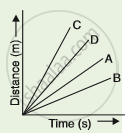# Four Cars A, B, C and D Are Moving on a Levelled, Straight Road. Their Distance-time Graphs Are Shown in the Given Figure. Which of the Following is the Correct Statement - Science

MCQ

Four cars A, B, C and D are moving on a levelled, straight road. Their distance-time graphs are shown in the given figure. Which of the following is the correct statement regarding the motion of these cars?#### Options

•  car A is faster than car D

• car B is the slowest

• car D is faster than the car C

• car C is the slowest

#### Solution

The slope of distance time graph gives the speed or the measure of rate of change of distance per unit time. So, the answer is,
(b) Car B is the slowest

Concept: Graphical Representation of Linear Motion - Displacement – Time Graph Or Distance – Time Graph
Is there an error in this question or solution?

#### APPEARS IN

Lakhmir Singh Science Class 9 Physics
Chapter 1 Motion
MCQ | Q 46 | Page 42